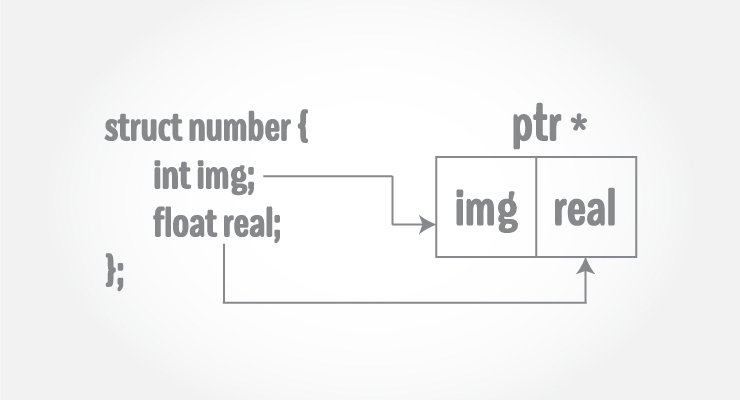C++ Pointers to StructureA pointer variable can be created not only for native types like (`int`, `float`, `double` etc.) but they can also be created for user defined types like structure.

If you do not know what pointers are, visit C++ pointers.

Here is how you can create pointer for structures:

``````#include <iostream>
using namespace std;

struct temp {
int i;
float f;
};

int main() {
temp *ptr;
return 0;
}``````

This program creates a pointer ptr of type structure temp.

Example: Pointers to Structure

``````#include <iostream>
using namespace std;

struct Distance
{
int feet;
float inch;
};

int main()
{
Distance *ptr, d;

ptr = &d;

cout << "Enter feet: ";
cin >> (*ptr).feet;
cout << "Enter inch: ";
cin >> (*ptr).inch;

cout << "Displaying information." << endl;
cout << "Distance = " << (*ptr).feet << " feet " << (*ptr).inch << " inches";

return 0;
}``````

Output

```Enter feet: 4
Enter inch: 3.5
Displaying information.
Distance = 4 feet 3.5 inches```

In this program, a pointer variable ptr and normal variable d of type structure Distance is defined.

The address of variable d is stored to pointer variable, that is, ptr is pointing to variable d. Then, the member function of variable d is accessed using pointer.

Note: Since pointer ptr is pointing to variable d in this program, `(*ptr).inch` and `d.inch` is exact same cell. Similarly, `(*ptr).feet` and `d.feet` is exact same cell.

The syntax to access member function using pointer is ugly and there is alternative notation -> which is more common.

```ptr->feet is same as (*ptr).feet
ptr->inch is same as (*ptr).inch```